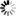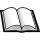Normal view

# An introduction to IoT analytics / Harry G. Perros.

Material type:TextSeries: Publisher: Boca Raton : CRC Press, 2021Edition: First editionDescription: 1 online resourceContent type:
• text
Media type:
• computer
Carrier type:
• online resource
ISBN:
• 9781000337860
• 9781003139041
Additional physical formats: Print version:: An introduction to IoT analyticsDDC classification:
• 004.67 PER  23
LOC classification:
• TK5105.8857
Contents:
Review of probability theory -- Simulation techniques -- Hypothesis testing -- Multivariable linear regression -- Time series forecasting -- Dimensionality reduction -- Clustering techniques -- Classification techniques -- Artificial neural networks -- Support vector machines -- Hidden Markov models.
Summary: "An Introduction to IoT Analytics covers techniques that can be used to analyze data from IoT sensors and also addresses questions regarding the performance of an IoT system. It strikes a balance between practice and theory so that one can learn how to apply these tools in practice with a good understanding of their inner workings. It is an introductory book for readers that have no familiarity with these techniques. The techniques presented in the book come from the areas of Machine Learning, Statistics, and Operations Research. Machine Learning techniques are described that can be used to analyze IoT data generated from sensors for clustering, classification, and regression. The statistical techniques described can be used to carry out regression and forecasting of IoT sensor data, and dimensionality reduction of data sets. Operations Research is concerned with the performance of an IoT system by constructing a model of a system under study, and then carry out what-if analysis. The book also describes simulation techniques. Key features: IoT analytics is not just Machine Learning but it also involves other tools, such as, forecasting and simulation techniques. Many diagrams and examples are given throughout the book to better explain the material presented. At the end of each chapter, there is a project designed to help the reader to better understand the techniques described in the chapter. The material is this book has been class tested over several semesters"-- Provided by publisher.
List(s) this item appears in: STEM & ENGINEERING CORNER
Tags from this library: No tags from this library for this title.
Star ratingsAverage rating: 0.0 (0 votes)
Holdings
Item type Current library Shelving location Call number Copy number Status Date due BarcodeBooks CamTech Library General Collections 004.67 PER (Browse shelf(Opens below)) C.1 Available CamTech 001088

Includes bibliographical references and index.

Review of probability theory -- Simulation techniques -- Hypothesis testing -- Multivariable linear regression -- Time series forecasting -- Dimensionality reduction -- Clustering techniques -- Classification techniques -- Artificial neural networks -- Support vector machines -- Hidden Markov models.

"An Introduction to IoT Analytics covers techniques that can be used to analyze data from IoT sensors and also addresses questions regarding the performance of an IoT system. It strikes a balance between practice and theory so that one can learn how to apply these tools in practice with a good understanding of their inner workings. It is an introductory book for readers that have no familiarity with these techniques. The techniques presented in the book come from the areas of Machine Learning, Statistics, and Operations Research. Machine Learning techniques are described that can be used to analyze IoT data generated from sensors for clustering, classification, and regression. The statistical techniques described can be used to carry out regression and forecasting of IoT sensor data, and dimensionality reduction of data sets. Operations Research is concerned with the performance of an IoT system by constructing a model of a system under study, and then carry out what-if analysis. The book also describes simulation techniques. Key features: IoT analytics is not just Machine Learning but it also involves other tools, such as, forecasting and simulation techniques. Many diagrams and examples are given throughout the book to better explain the material presented. At the end of each chapter, there is a project designed to help the reader to better understand the techniques described in the chapter. The material is this book has been class tested over several semesters"-- Provided by publisher.

Description based on print version record and CIP data provided by publisher.

There are no comments on this title.